Get instant live expert help with Excel or Google Sheets“My Excelchat expert helped me in less than 20 minutes, saving me what would have been 5 hours of work!”

#### Post your problem and you’ll get Expert help in seconds.

Your message must be at least 40 characters
Our professional Expert are available now. Your privacy is guaranteed.

# How to Use Absolute and Relative Reference in Excel and Google Sheets on Mac

The dollar sign (\$) in Excel tells Excel to change or not to change a cell reference when a formula is copied to another cell. This tutorial will guide all levels of Excel users on how to manipulate absolute and relative references in Excel and Mac.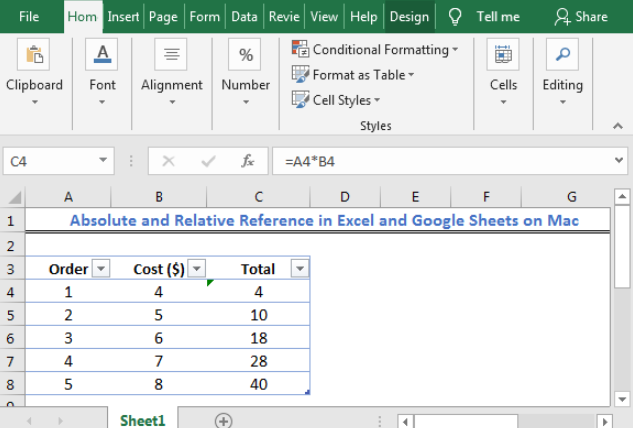Figure 1: Absolute and Relative Reference in Excel and Google Sheets on Mac

*** To copy down a formula, click on the cell with the formula, at the bottom right, you will see a small box. Take your cursor to the box. Now you will see a + sign. Left-click your mouse and drag down the + sign.

## What is a Relative Cell Reference in Excel?

A relative cell reference is Excel is a Cell address without the dollar sign in the row and column as we copy down the formula. As we copy down a formula with a relative cell reference, the cell reference changes based on the rows and columns relative positions.

## How to Use Relative Reference in Excel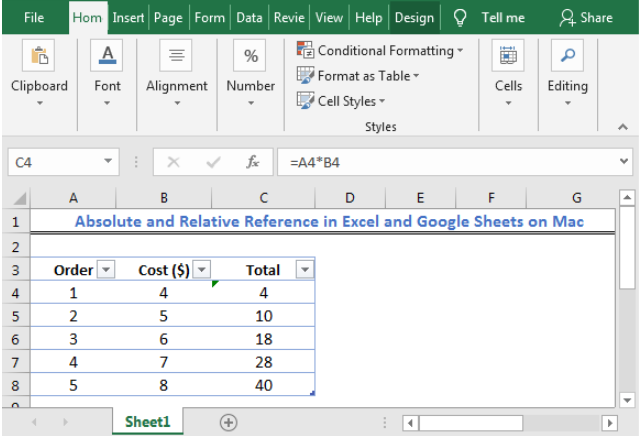Figure 2: Relative Cell reference in Excel

As shown in figure 2, the returned result in Cell C4 is for the multiplied values referenced in Cell A4 and Cell B4. The formula looks like this:

Cell D4: `=A4*B4`

Cell D5: `=A5*B5`

Cell D6: `=A6*B6`

Cell D7: `=A7*B7`

Cell D8: `=A8*B8`

What is an Absolute Reference?

An absolute cell reference is Excel is a Cell address with the dollar sign in the row and column as we copy down the formula. As we copy down a formula with an absolute cell reference, the cell reference remains unchanged regardless of the row and/or column.

How to Do Absolute Reference in Excel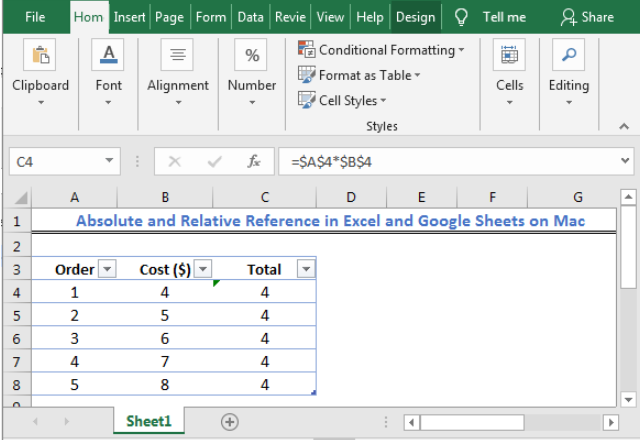Figure 3: Absolute Cell reference in Excel

As shown in figure 3, the returned result in Cell C4 is for the absolute multiplied values referenced in Cell A4 and Cell B4. The formula looks like this when copied down:

Cell D4: `=\$A\$4*\$B\$4`

Cell D5: `=\$A\$4*\$B\$4`

Cell D6: `=\$A\$4*\$B\$4`

Cell D7: `=\$A\$4*\$B\$4`

Cell D8: `=\$A\$4*\$B\$4`

## What is Mixed (Cell) Reference?

A mixed cell reference in Excel is a Cell address that has either the column letter or row number fixed. This means that either the column letter or row number is locked or absolute referenced, e.g. in \$A1, Column A is locked but row 1 is relative.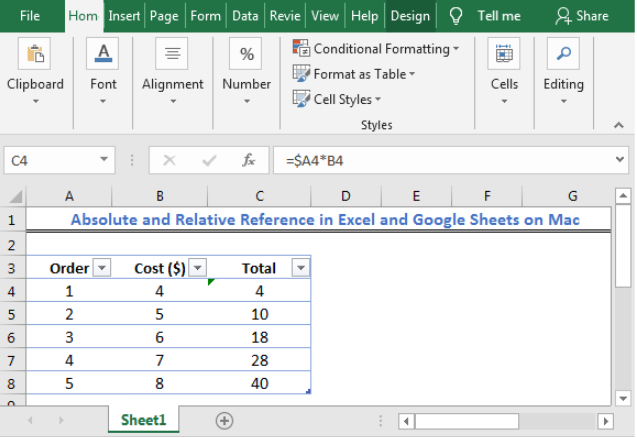Figure 4a: Mixed Cell reference in Excel

As shown in figure 4a, the returned result in Cell C4 is for the multiplied values with a mixed column (Column A) reference in Cell A4 and Cell B4. The formula looks like this when copied down:

Cell D4: `=\$A4*B4`

Cell D5: `=\$A5*B5`

Cell D6: `=\$A6*B6`

Cell D7: `=\$A7*B7`

Cell D8: `=\$A8*B8`

If we add the dollar sign to the row number of Column A, the result will look like this: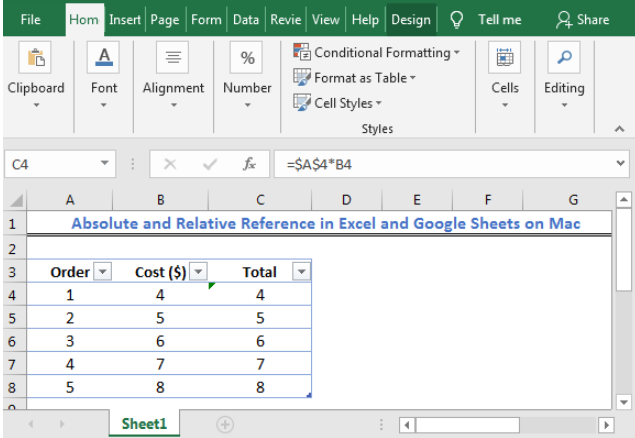Figure 4b: Mixed Cell reference in Excel

As shown in figure 4b, the returned result in Cell C4 is for the multiplied values with a locked reference for Column A and Row 4 in Cell A4 and Cell B4. The formula looks like this when copied down:

Cell D4: `=\$A\$4*B4`

Cell D5: `=\$A\$4*B5`

Cell D6: `=\$A\$4*B6`

Cell D7: `=\$A\$4*B7`

Cell D8: `=\$A\$4*B8`

In the example below, we will see that although Column B is a relative reference, because Row 4 is locked in the formula, our result is always returned as 4.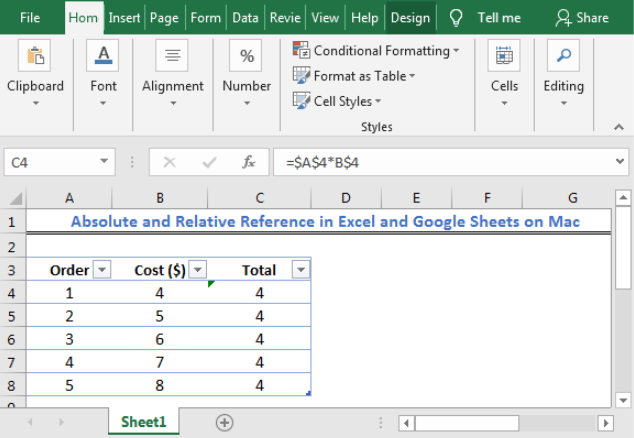Figure 4c: Mixed Cell reference in Excel

The formula in figure 4c remains \$A\$4*B\$4 when copied down.

## Shortcut to Switch between Mixed, Absolute and Relative References

1. We will select the cell with our formula, e.g. Cell C4 in Figure 2
2. We will press the F2 KEY to enter edit mode. We can also double-click the cell
3. We will select the Cell reference we want to change
4. We will press the F4 KEY to toggle between the four types of cell references.

Note: Pressing the F4 key without selecting any cell reference causes the reference to the left of the mouse pointer to be automatically selected. This results in a change to another reference type.

## Shortcut to Switch between Mixed, Absolute and Relative References for Mac

For Mac, we can use the shortcuts below:

T

fn + F4

## Instant Connection to an Excel Expert

Most of the time, the problem you will need to solve will be more complex than a simple application of a formula or function. If you want to save hours of research and frustration, try our live Excelchat service! Our Excel Experts are available 24/7 to answer any Excel question you may have. We guarantee a connection within 30 seconds and a customized solution within 20 minutes.